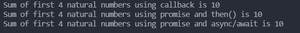# How to avoid callback hell in Node.js ?

• Last Updated : 30 Sep, 2021

Callback hell in Node.js is the situation in which we have complex nested callbacks. In this, each callback takes arguments that have been obtained as a result of previous callbacks. This kind of callback structure leads to lesser code readability and maintainability.

We can avoid the callback hell with the help of Promises. Promises in javascript are a way to handle asynchronous operations in Node.js. It allows us to return a value from an asynchronous function like synchronous functions. When we return something from an asynchronous method it returns a promise which can be used to consume the final value when it is available in the future with the help of then() method or await inside of async functions. The syntax to create a promise is mentioned below.

```const promise = new Promise(function(resolve, reject){
// code logic
});```

Example: In the code example mentioned below, we simulate an asynchronous add operation with the help of setTimeout()

• First, we create an add() function that takes three arguments in which two are the numbers that we want to add and the third one is the callback function which is called with the result of add operations after 2 seconds. Then, we calculate the result of the addition of the first four natural numbers using a nested callback to simulate a callback hell.
• After that, we create an addPromise() function that returns a promise and this promise is resolved after two seconds of calling the function. Then we consume the promise using the then() method and async/await.

## Javascript

 `// The callback function for function ` `// is executed after two seconds with ` `// the result of addition ` `const add = ``function` `(a, b, callback) { ` `  ``setTimeout(() => { ` `    ``callback(a + b); ` `  ``}, 2000); ` `}; ` ` `  `// Using nested callbacks to calculate ` `// the sum of first four natural numbers. ` `add(1, 2, (sum1) => { ` `  ``add(3, sum1, (sum2) => { ` `    ``add(4, sum2, (sum3) => { ` `      ``console.log(`Sum of first 4 natural  ` `      ``numbers using callback is \${sum3}`); ` `    ``}); ` `  ``}); ` `}); ` ` `  `// This function returns a promise ` `// that will later be consumed to get ` `// the result of addition ` `const addPromise = ``function` `(a, b) { ` `  ``return` `new` `Promise((resolve, reject) => { ` `    ``setTimeout(() => { ` `      ``resolve(a + b); ` `    ``}, 2000); ` `  ``}); ` `}; ` ` `  `// Consuming promises with the chaining of then() ` `// method and calculating the result ` `addPromise(1, 2) ` `  ``.then((sum1) => { ` `    ``return` `addPromise(3, sum1); ` `  ``}) ` `  ``.then((sum2) => { ` `    ``return` `addPromise(4, sum2); ` `  ``}) ` `  ``.then((sum3) => { ` `    ``console.log( ` `      ```Sum of first 4 natural numbers using  ` `       ``promise and then() is \${sum3}` ` `    ``); ` `  ``}); ` ` `  `// Calculation the result of addition by ` `// consuming the promise using async/await ` `(async () => { ` `  ``const sum1 = await addPromise(1, 2); ` `  ``const sum2 = await addPromise(3, sum1); ` `  ``const sum3 = await addPromise(4, sum2); ` ` `  `  ``console.log( ` `    ```Sum of first 4 natural numbers using  ` `     ``promise and async/await is \${sum3}` ` `  ``); ` `})(); `

Output:My Personal Notes arrow_drop_up
Recommended Articles
Page :